# ISEE Middle Level Math : How to find the part from the whole

## Example Questions

1 2 3 4 5 7 Next →

### Example Question #61 : How To Find The Part From The Whole

What isof?Explanation:

To find a fraction of a whole number, we will multiply the fraction by the whole number. So, we get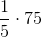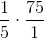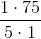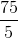### Example Question #62 : How To Find The Part From The Whole

What is a third of 27?Explanation:

To find a fraction of a whole number, we will multiply the fraction by the whole number.

We know a third is the same as.  So, we get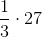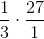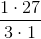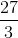1 2 3 4 5 7 Next →

### All ISEE Middle Level Math Resources